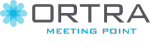# THE STOCHASTIC TEST COLLECTION PROBLEM: A MODEL AND SOLUTION APPROACHES

author.DisplayName author.DisplayName author.DisplayName
Industrial Engineering, Tel-Aviv University, ישראל

Health care systems invest a substantial amount of efforts to diagnose a patient. Potential tests can be done for this purpose, where each test is associated with a certain cost. A major task is to select a subset of tests out of a potentially very large (and expensive) set. This raises a new challenging bi-objective optimization problem. The objectives are (1) minimizing the expected cost associated with classification errors, i.e., diagnosing A while the true diagnosis is B; (2) minimizing the total cost of the tests.

Let us define the Stochastic Test Collection Problem (STCP). The inputs for our model consists of the following: (a) a set of potential tests, where each test having an associated test cost; (b) a list of all possible readings of results from the tests; (c) each reading is associated probabilistically with several diagnoses; (d) a classification error cost defined for each pair of different diagnoses, which represents the cost associated with a wrong classification. A solution to the STCP is a subset of tests along with a mapping of each possible signature (in the collection of all partial readings induced by the selected tests) to diagnosis. The tradeoff between the two objectives is clear since performing more tests and/or more expensive ones is likely to reduce the expected cost of classification errors but increase the total cost of the tests. The challenge (and the interest) of the problem stems from the fact that the diagnosis can rarely be inferred from the result of a single test. Instead, it is concluded from a combination of many results (signature) of different tests. Moreover, these results can be affected by random noise.

The considered problem is a generalization of the minimum test collection problem (TCP). The decision version of this problem known to be NP-Complete and it is also known to be APX-Hard. The special case of the TCP, using our terminology, is the case when all the tests can produce binary results; each reading is associated with a unique binary diagnosis (“positive” or “negative"), and the cost of all the tests are identical (one unit without loss of generality).

We apply three metaheuristic methods for the STCP, namely tabu search (TS), cross-entropy (CE), and binary gravitational search algorithm (BGSA). We also solve small instances of the problem by enumerating all the possible subsets of tests and evaluate their solutions in order to create a benchmark for our heuristic. We use these solutions to demonstrate the effectiveness of our methods. The TS delivers the best solutions for the larger instances given a reasonable budget of computational efforts.

החברה המארגנת: ארטרא בע"מ, רחוב יגאל אלון 94 תל אביב 6109202 טלפון: 03-6384444, פקס: 6384455–03
iem@ortra.com מייל לשאלות